# Sampling Edges and More

### Amherst College

Based on joint work withTalya Eden
MIT/BUDana Ron
Tel Aviv University

## Outline

1. Introductory Remarks
2. Sampling Edges: A Simple Algorithm
3. Optimality?
4. Parameterization by Arboricity
5. Applications and Open Questions

# 1. Introductory Remarks

Assistant Professor in CS at Amherst College

Previously:

• Postdocs at MPI for Informatics in Saarbrücken, Germany and Tel Aviv University

Generally interested in CS Theory: algorithms and complexity

• role of communication and locality in algorithms
• local and distributed algorithms
• sub-linear algorithms
• algorithms with uncertainty about input

## A Large(?) Graph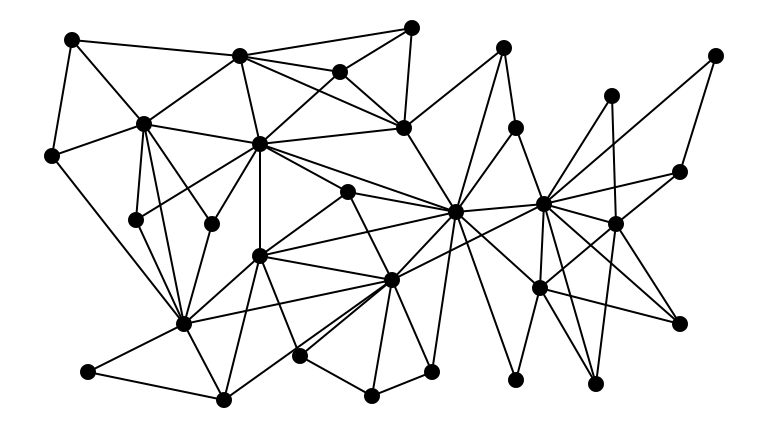## Goal: Sample a Random Edge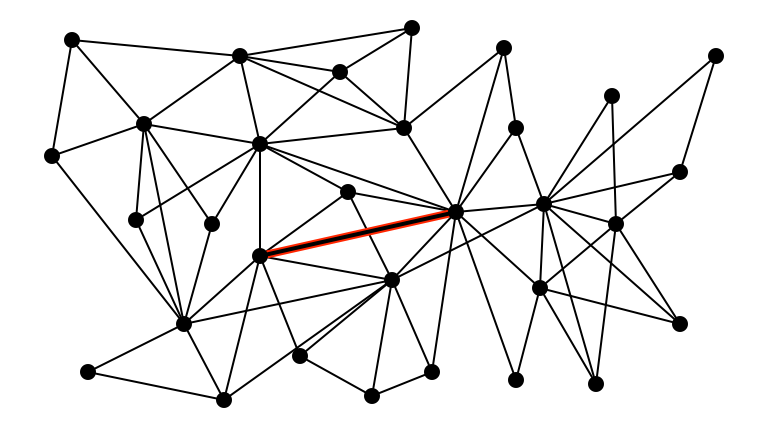## Problem: Don’t Initially Know Edges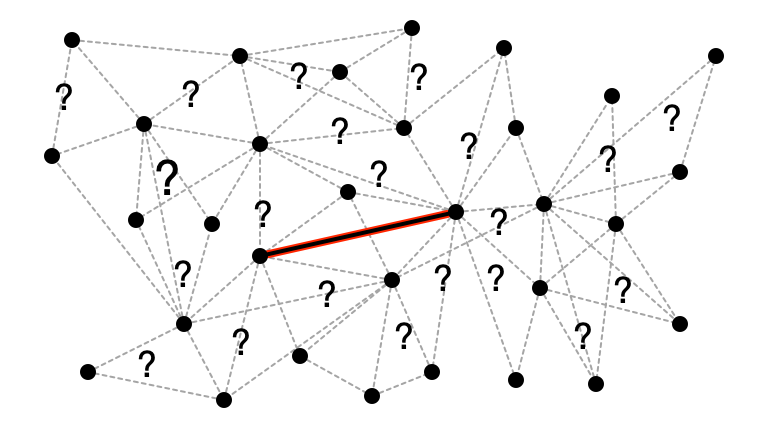## Computational Model

• $G = (V, E)$ a very large graph
• access to $G$ provided via queries:
• sample a (uniformly random) vertex $v \in V$
• degree query: return the degree of vertex, $d(v)$
• neighbor query: return the $i$-th neighbor of $v$
• pair query: return whether or not $(u, v) \in E$

A.k.a. general graph model in property testing

## Problem Statement

Goal. Sample an edge $e \in E$ from an almost uniform distribution.

• use as few queries as possible

Point-wise Uniformity. Want every edge $e$ to be sampled with probability

• $\Pr(e \text{ is sampled}) = \frac{(1 \pm \varepsilon)}{m}$

where $m$ is number of edges.

# 2. Sampling Edges: A Simple Algorithm

## Warm-Up

What if an upper bound $\Delta$ on maximum degree is known?

1. pick vertex $v$ uniformly at random
2. pick a number $i$ from ${1, 2, \ldots, \Delta}$ uniformly at random
3. query $i$th neighbor of $v$
• if a vertex $w$ is found, return $e = (v, w)$
• otherwise, go to step 1

## A Graph with $\Delta = 4$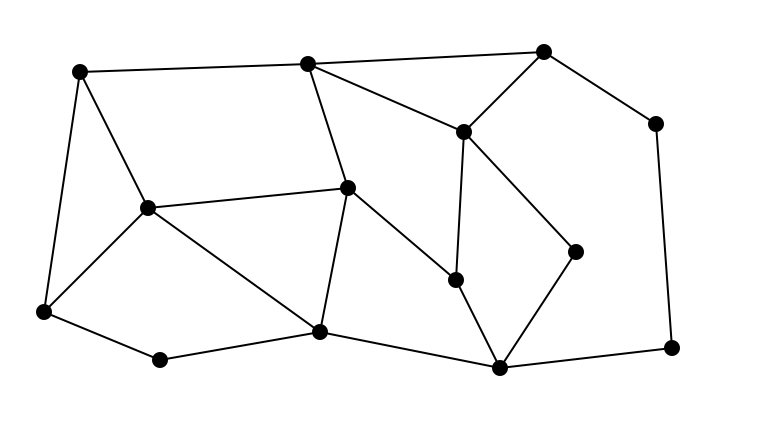## Pick a Random Vertex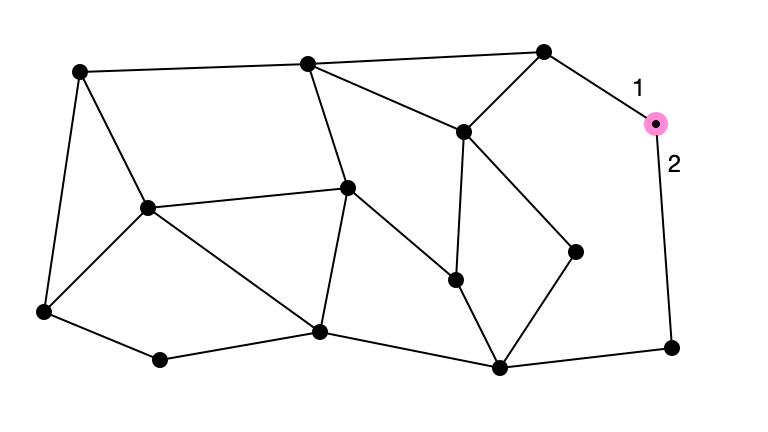## Pick a Random Number $$\leq \Delta$$: 3## Failure. Try Again!## Pick a Random Vertex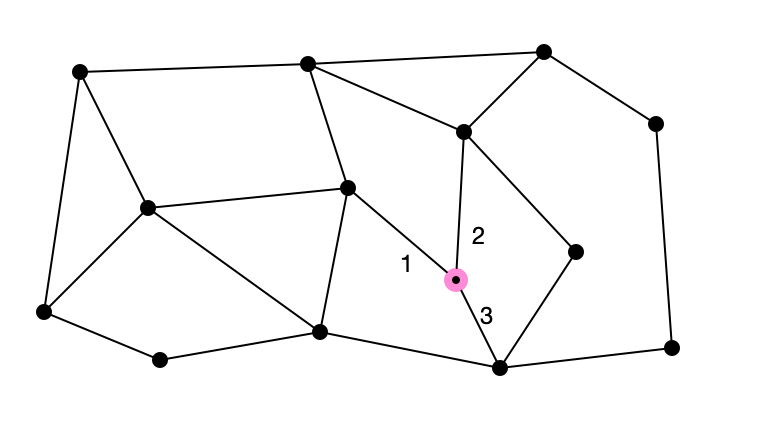## Pick a Random Number $\leq \Delta$: 2## Return Selected Edge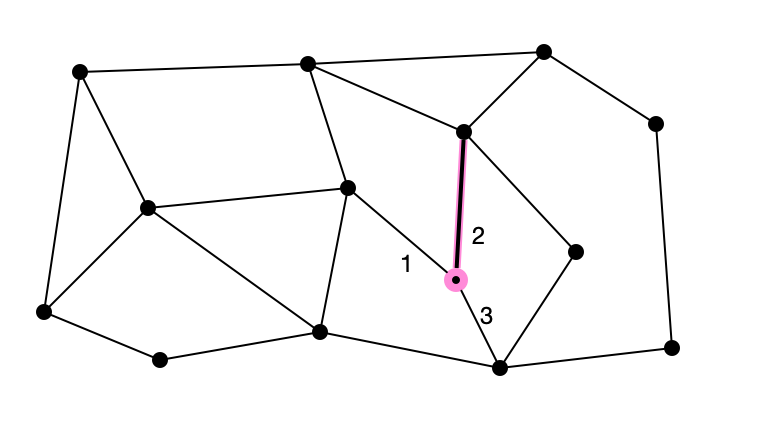## Simple Algorithm

What if an upper bound $\Delta$ on maximum degree is known?

1. pick vertex $v$ uniformly at random
2. pick a number $i$ from ${1, 2, \ldots, \Delta}$ uniformly at random
3. query $i$th neighbor of $v$
• if a vertex $w$ is found, return $e = (v, w)$
• otherwise, go to step 1

Claim 1. Each edge sampled with probability $\frac{2}{n \cdot \Delta}$.

Claim 2. Expected number of queries until success is $O\left(\frac{n \cdot \Delta}{m}\right)$.

## Question 1

Claim 2. Expected number of queries until success is $O\left(\frac{n \cdot \Delta}{m}\right)$.

For what graphs is this algorithm efficient?

## Question 2

Claim 2. Expected number of queries until success is $O\left(\frac{n \cdot \Delta}{m}\right)$.

What if there is no (known) upper bound on maximum degree?

## More Questions

Can we do better…

…when no bound on $\Delta$ is known?

…when $n \Delta / m$ is large?

Main Question. For what (families of) graphs can we sample edges efficiently?

• What promises ensure efficient edge sampling?

## An Illustrative Example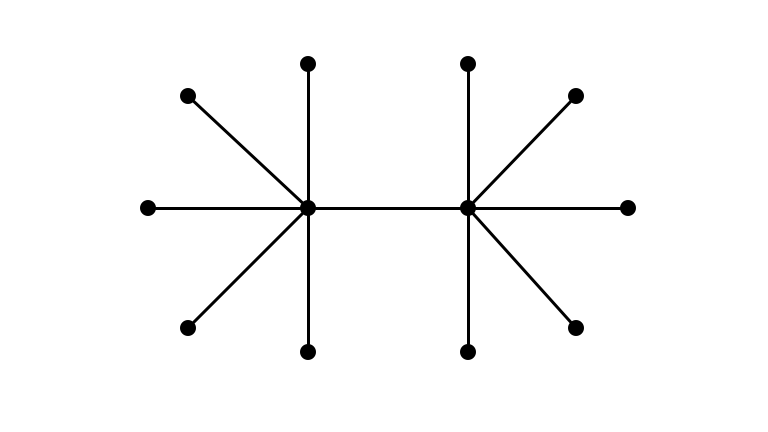## Observe• $\Delta = \Theta(n)$
• $m = \Theta(n)$
• $\implies n \Delta / m = \Theta(n)$
• can always sample with $\Theta(n)$ queries
• get degrees of all vertices

## Leaf Edges are Easy to Sample## See Middle Edge w/ Prob $$\Omega(1/n)$$?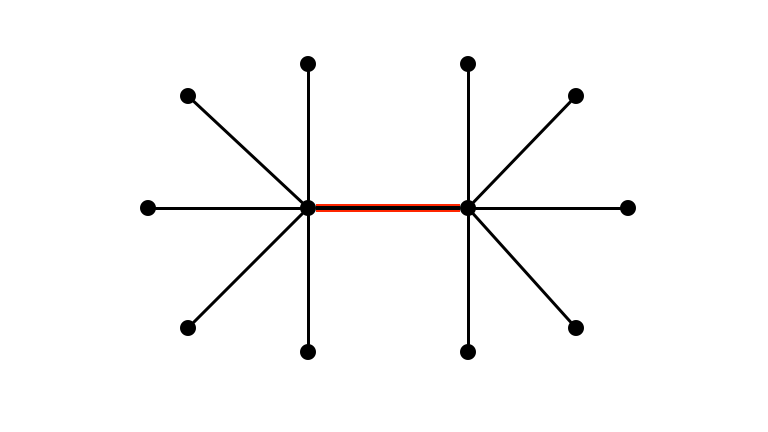## Access Middle Vertices via Neighbors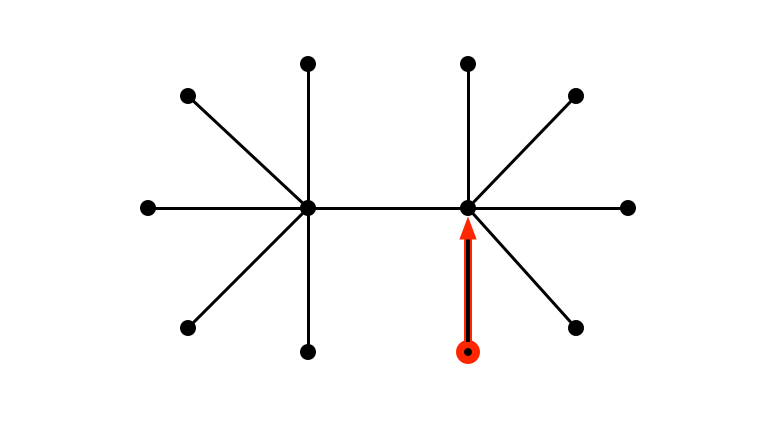## Access Middle Vertices via Neighbors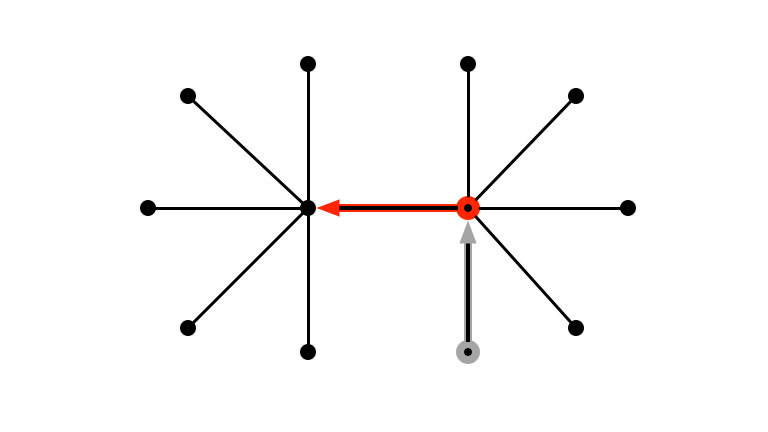## Generalizing the Procedure

• partition vertices according to degree
• use threshold $\theta$
• if $d(v) \leq \theta$, $v$ is light
• if $d(v) > \theta$, $v$ is heavy
• sample edges from light vertices as in warm-up
• to sample edges from heavy vertices:
• sample an edge $(u, v)$ with $u$ light
• if $v$ is heavy, pick a random neighbor $w$
• return $(v, w)$

## Technicalities## Edges $$\to$$ Oriented Pair of Edges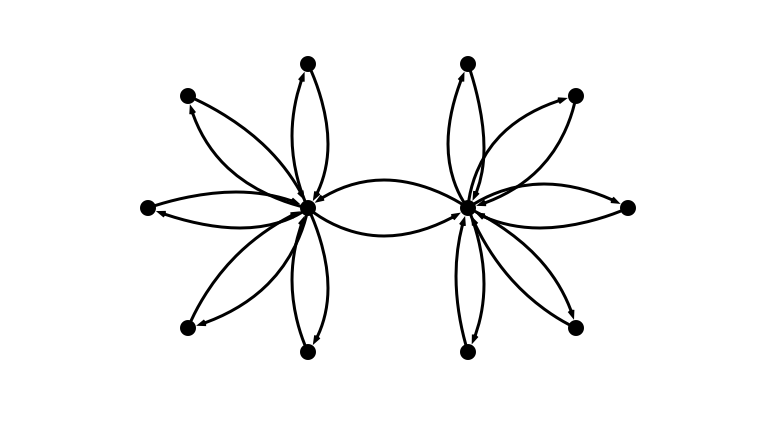## Partition Vertices into Heavy/Light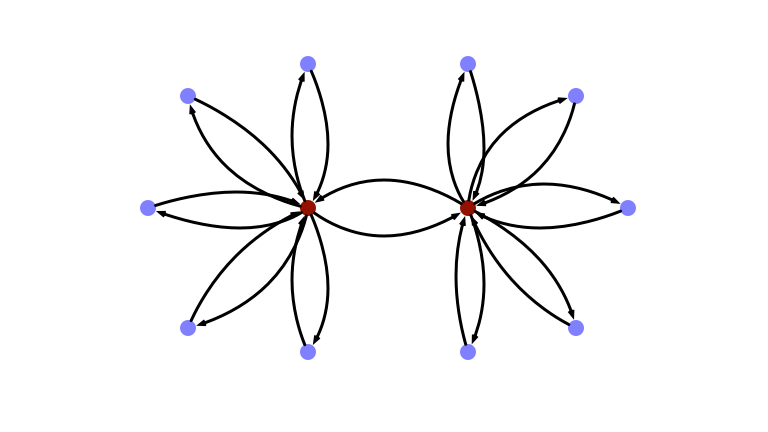## Partition Edges into Heavy/Light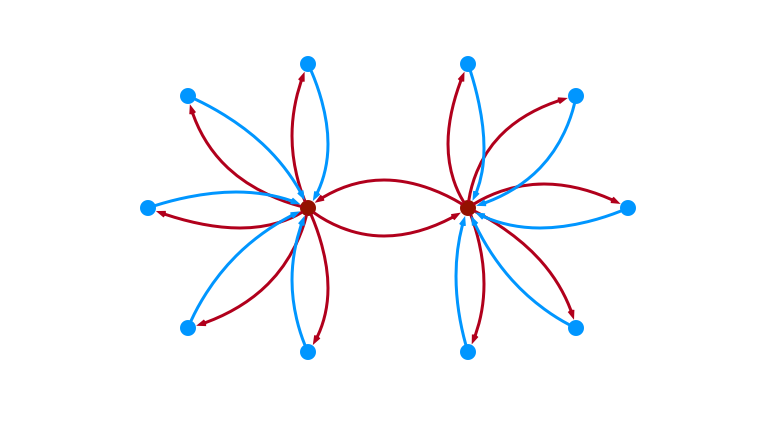## Another View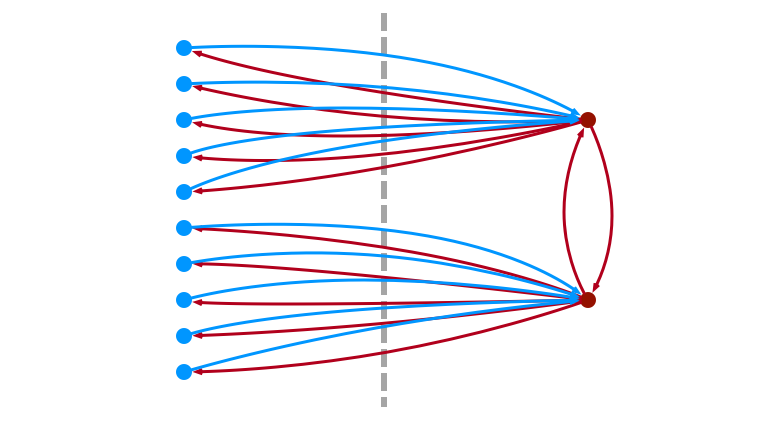## To Sample Light Edge## 1. Sample Vertex; Check Light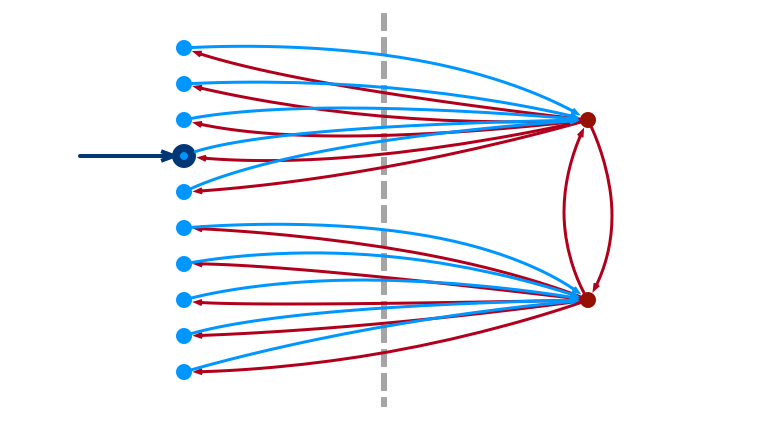## 2. Pick Random $$i \leq \theta$$## 3. Return $$i$$th Edge (or Fail)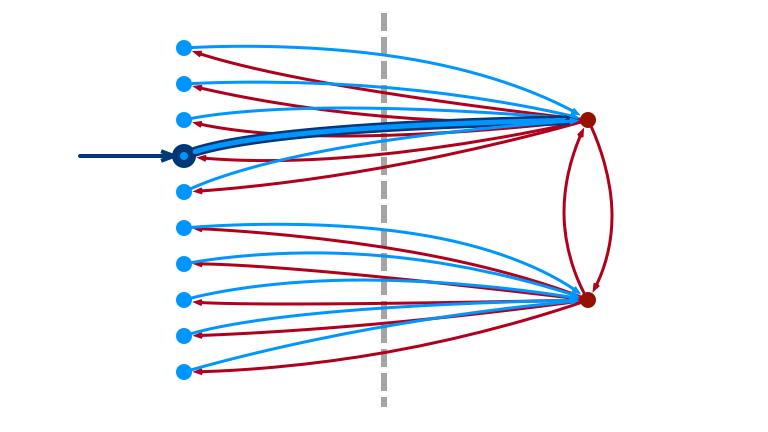## To Sample Heavy Edge## 1. Sample Light Edge $$(u, v)$$## 2. If $$v$$ is Light, Fail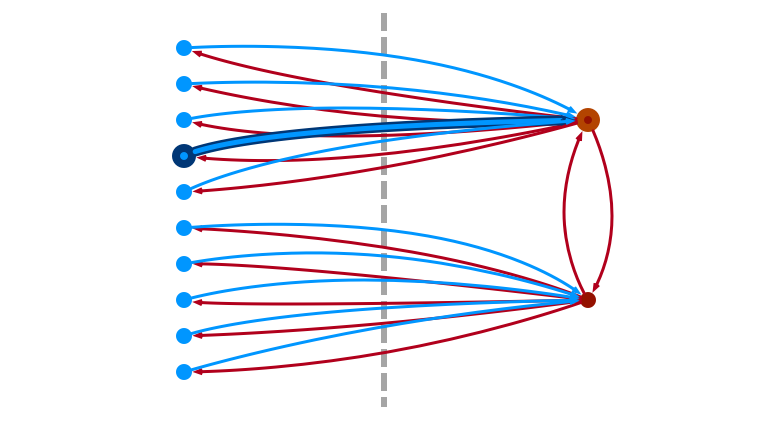## 3. Otherwise, Pick Random Neighbor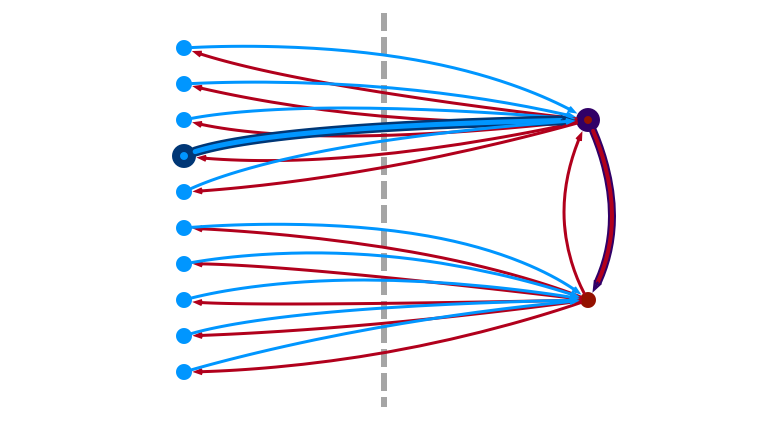## Choosing Threshold $$\theta$$

Want:

1. $\theta$ as small as possible
• want light edge sampling to succeed
2. every heavy vertex has mostly light neighbors
• can reach every heavy vertex in one step from light
• hit each heavy vertex with prob. proportional to its degree

## A Good $\theta$

Choose:

• $\theta = \sqrt{m / \varepsilon}$
• $m$ is number of oriented edges

Lemma. Heavy vertices have at most $\varepsilon$ fraction of heavy neighbors.

Proof. Suppose $> \varepsilon$ fraction:

• $> \varepsilon \cdot (\sqrt{m / \varepsilon}) = \sqrt{\varepsilon m}$ heavy neighbors
• each neighbor has degree $> \sqrt{m / \varepsilon}$
• $> m$ edges $$\Rightarrow\!\Leftarrow$$

## An Issue?

We chose $\theta = \sqrt{m / \varepsilon}$

What is the problem with this?

• How do we know $m$!?

We can get a good enough estimate of $m$ with $O(n / \sqrt{m})$ queries:

• Feige (2006)
• Goldriech & Ron (2008)

## The General Algorithm

Until an edge is sampled, flip a coin

• with probability 1/2, try to sample light edge
• pick $u$ uniformly at random
• if $u$ is light, pick random $i \leq \theta$ u.a.r.
• if $i \leq d(u)$, return $(u, v)$ ($v$ is $i$th neighbor)
• with probability 1/2, try to sample heavy edge
• try to sample light edge $(u, v)$
• if $v$ is heavy, return random incident edge $(v, w)$

## Analysis

Claim 1. Light edge procedure returns every light edge with probability $\frac{1}{n \cdot \theta}$. $\Box$

Claim 2. Heavy edge procedure returns every heavy edge $e$ with probability $p(e)$ satisfying

• $\frac{1 - \varepsilon}{n \cdot \theta} \leq p(e) \leq \frac{1}{n\cdot\theta}$.

## Proof of Claim 2.

Suppose $v$ is a heavy vertex, $d_L(v)$ the number of its light neighbors.

By Lemma (and choice of $\theta$), $d_L(v) \geq (1 - \varepsilon) d(v)$

By Claim 1, the probability that some $(u, v)$ is returned in light edge sample is

• $$\frac{d_L(v)}{n \theta} \geq (1 - \varepsilon) \frac{d(v)}{n \theta}$$ in which case run succeeds.

The probability that heavy sample returns $(v, w)$ is then

• $\frac{d_L(v)}{n \theta} \cdot \frac{1}{d(v)} \geq (1 - \varepsilon) \frac{1}{n \theta}.$

## Running Time

• probability of success is at least
• $\frac{(1 - \varepsilon) m}{n \cdot \theta} \geq \frac{\sqrt{\varepsilon m}}{2 n}$
• number of trials until success is $O\left(\frac{n}{\sqrt{\varepsilon m}}\right)$

## Main Result

Theorem. The general algorithm samples edges almost uniformly using $O\left(\frac{n}{\sqrt{\varepsilon m}}\right)$ queries in expectation.

# 3. Optimality?

## Can We Do Better?

Question. Can we sample edges using $o\left(\frac{n}{\sqrt{m}}\right)$ queries?

## No!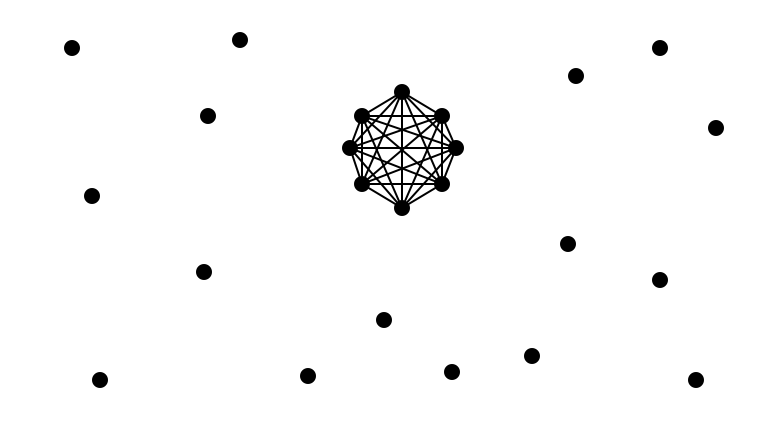## A Lower Bound

Theorem. Any algorithm that samples edges almost uniformly requires $$\Omega\left(\frac{n}{\sqrt{m}}\right)$$ queries.

Eh, maybe not?

## Easy Instance ($n = 24, m = 44$)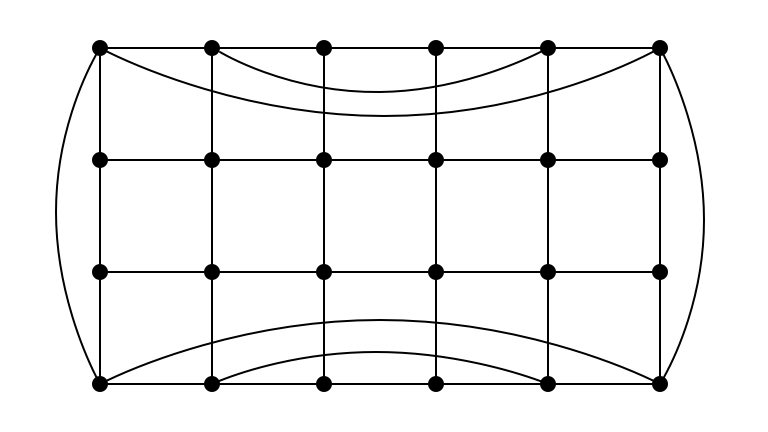## Hard Instance ($n = 24, m = 44$)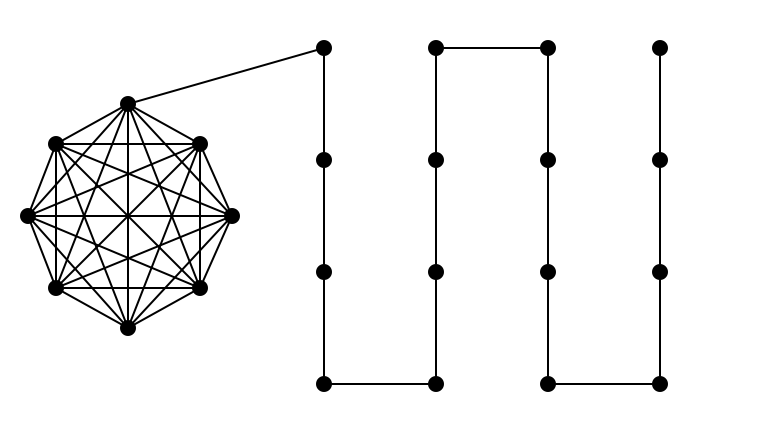## What Makes an Instance Hard?

Having large proportion of edges hidden among a small proportion of vertices!

• if no such dense subgraphs, maybe we can sample more efficiently…

# 4. Parameterization by Arboricity

## What is Arboricity?

The arboricity of a graph $G$, denoted $\alpha(G)$ is:

1. the maximum average degree of any subgraph of $G$

2. the minimum number of spanning trees (forests) whose union is $G$

Theorem (Nash-Williams, 1964). The two definitions above are equivalent.

## High $\Delta$, Small $\alpha$## High $\Delta$, Small $\alpha$, 1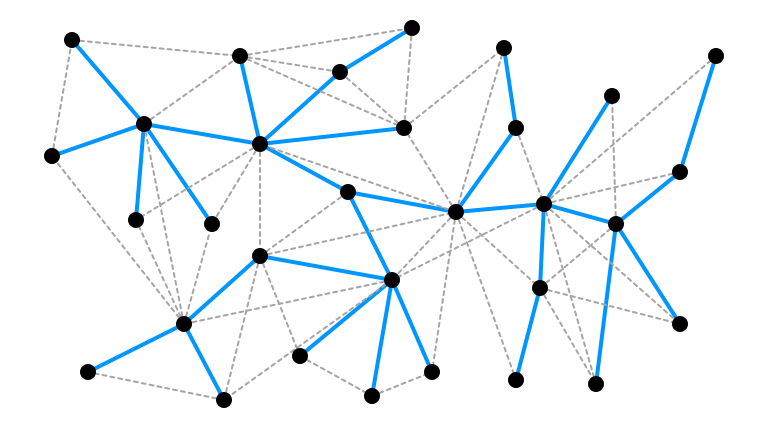## High $\Delta$, Small $\alpha$, 2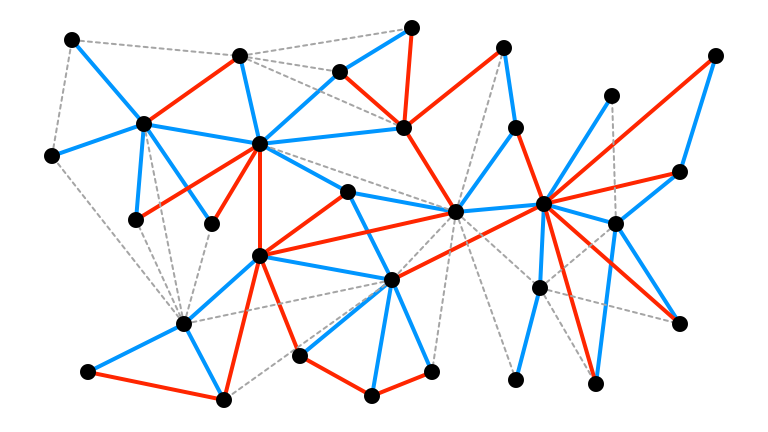## High $\Delta$, Small $\alpha$, 3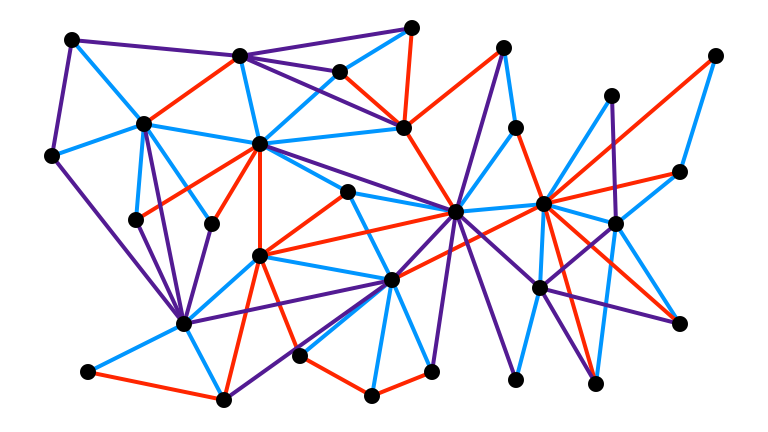## Does Knowing Arboricity Help?

Intuition. If $\alpha \approx \frac{m}{n}$, then no subgraphs much denser than average

• no place to hide edges

But how to use this fact?

• before, our tricky instance was a tree—$\alpha = 1$…

## Structural Lemma

Lemma. If $G = (V, E)$ has arboricity at most $\alpha$, then $V$ can be partitioned into layers $L_0, L_1, \ldots, L_\ell$ with $\ell \leq \log n$ such that:

1. $v \in L_0 \iff v$ has degree at most $O(\alpha \log n)$,

2. every $v \in L_i$ with $i \geq 1$ has $1 - O(1/\log n)$ fraction of neighbors in $L_0, L_1, \ldots, L_{i-1}$.

## Structural Lemma, Illustrated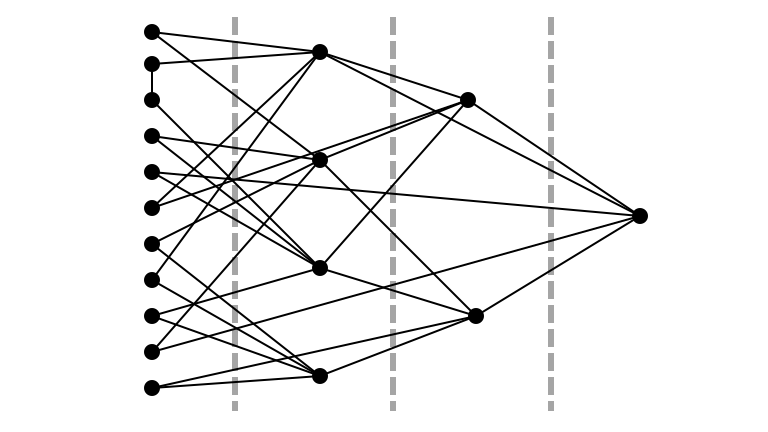## Observation

Layered decomposition $\approx$ light/heavy decomposition from before

• can sample “light” edges (from $L_0$) easily
• to access heavy edges, start at $L_0$ and walk upwards
• all vertices reachable after $\leq \log n$ steps

## An Algorithm

Repeat until successful:

1. use rejection sampling to sampling to sample a vertex $u \in L_0$ with probability proportional to its degree
• note $u \in L_0 \iff d(u) \leq C \alpha \log n$
2. Pick $k \in {0,1,\ldots,\ell}$ uniformly at random.
3. Perform a simple random walk of length $k$ starting from $u$.
4. Abort if another vertex in $L_0$ is encountered.
5. If random walk ends at $v$, return $(v,w)$, where $w$ is a uniformly random neighbor of $v$.

## Illustration## Pick Random $u$ in $L_0$ Prop. to $d(u)$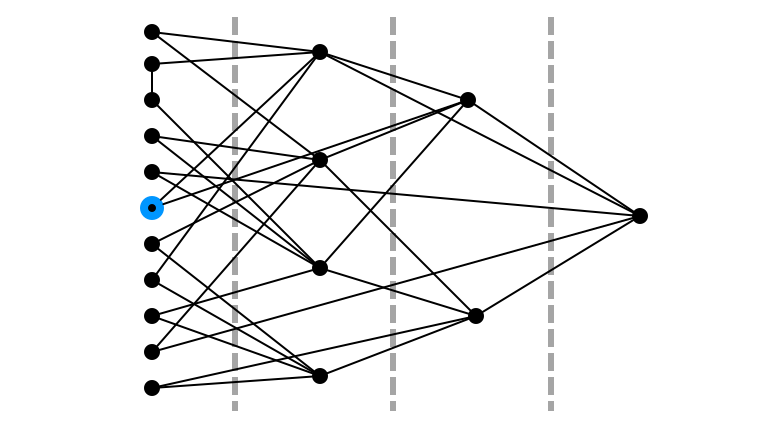## Pick Random $k$ in $\{0, 1, 2, 3\}$: 2## Random Walk Step $1$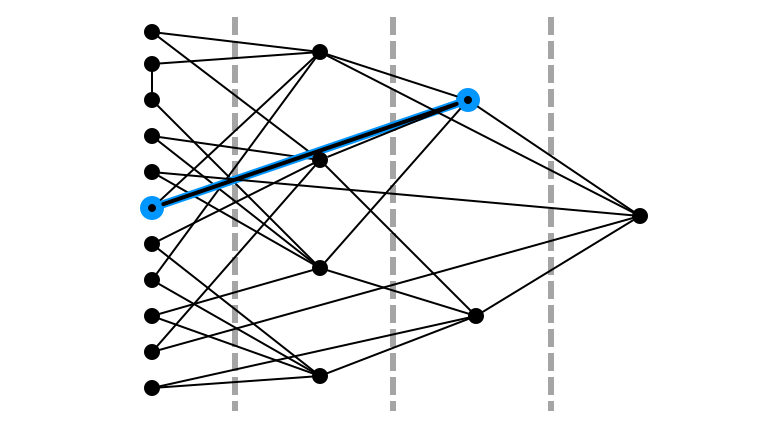## Random Walk Step $2$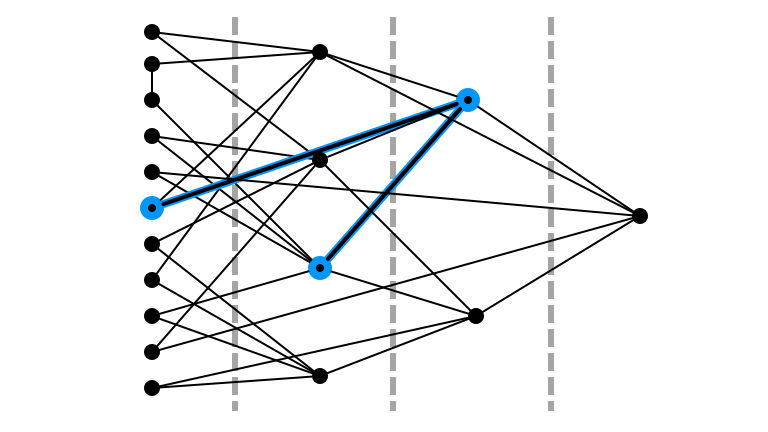## Didn’t Return to $L_0$## Return Random Incident Edge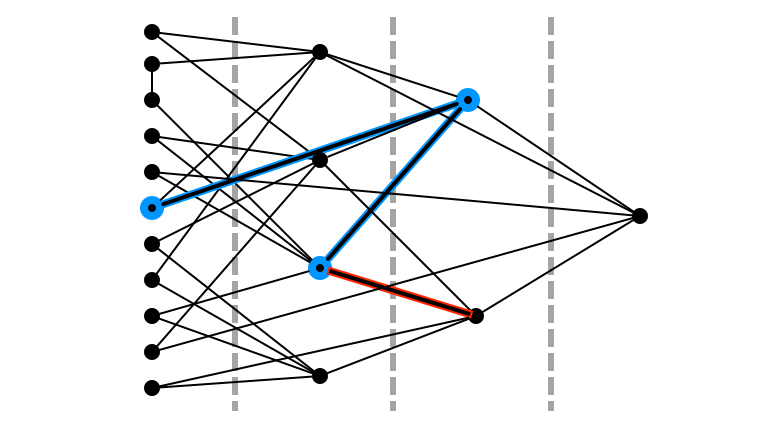## Main Result

Theorem. A single iteration returns each edge $e \in E$ with probability $p(e)$ satisfying

• $$(1 - \varepsilon) \frac{\varepsilon}{\alpha n \log^2 n} \leq p(e) \leq \frac{\varepsilon}{\alpha n \log^2 n}$$.

Therefore, the procedure samples edges almost uniformly using $O^*\left(\frac{\alpha n}{m}\right)$ queries in expectation.

Note. The quantity $\frac{\alpha n}{m}$ is precisely the ratio of maximum average degree of a subgraph ($\alpha$) to overall average degree ($m / n$).

• this measures “how hidden” a large collection of edges can be

## Interesting Feature

Observation. The layered decomposition doesn’t appear in the sampling algorithm!

• used to justify the algorithm
• provides intuition about the random walk process
• decomposition appears in the analysis of the algorithm

# 5. Applications and Open Questions

## Application of Technique: Sampling Cliques

What if we want to sample bigger subgraphs…not just single edges?

• E.g., sampling random triangles, 4-cliques, $k$-cliques

## Application of Technique: Sampling Cliques

• Define auxuiliary graph $H_{k}$:
• vertices in $H_{k}$ are $k-1$ cliques
• when vertices in $H_k$ share an edge, edge corresponds to $k$-clique
• sampling edges in $H_k$ corresponds to sampling $k$-cliques in $G$
• If $G$ has arboricity at most $\alpha$, then so does $H_k$
• Using arboricity algorithm to sample edges $H_k$
• to get vertices in $H_k$, must sample edges in $H_{k-1}$
• recursively call procedure in $H_{k-1}$

## Application of Technique: Sampling Cliques

Theorem. Almost uniformly random $k$-cliques can be sampled using $O^*\left(\frac{n \alpha^{k-1}}{n_k}\right)$ queries in expectation, where $n_k$ is the number of $k$-cliques in the graph.

• Upper bound is tight for wide range of parameters

Note. For $k > 2$, lower bound separates complexity of sampling and approximate counting!

• In bounded arboricity graphs, triangles can be approximately counted in $O^*(1)$ queries

## Black Box Applications

Previous works employ edge samples for other tasks:

• Counting arbitrary graphs

• Sampling arbitrary graphs

## Open Questions and Future Directions

1. Can we sample graphs other than cliques efficiently in low-arboricity graphs?

2. When do approximate queries suffice?
• e.g., almost uniformly random edges instead of exactly uniform
3. What general relationships can we find between different query models?
• recent papers introduce more exotic queries: IS, BIS, Inner Product,…
• how can we understand the relative computational power of these primitives?